# 3D Numerical Simulation and Influencing Factors of Loose Top Coal spontaneous Combustion in Roadway

3D Numerical Simulation and Influencing Factors of Loose Top Coal spontaneous Combustion in Roadway

Hongfen ZhangErxin Gao

China University of Mining & Technology, Beijing 100083, China

Hebei University of Engineering, Handan Hebei 056038, China

Corresponding Author Email:
zhanghongfenkd@sina.com
Page:
91-96
|
DOI:
http://doi.org/10.18280/ijht.330313
N/A
|
Accepted:
N/A
|
Published:
30 September 2015
| Citation

OPEN ACCESS

Abstract:

The spontaneous combustion of coal has a serious effect. To study the spontaneous combustion of the loose top coal in the roadway and its influencing factors, the 3D multi field coupling model including coal  and roadway is built.  The transient  law of the flow field, the temperature field and the mass transfer field at any moment during coal self-heating process is acquired by COMSOL numerical simulation. On y=0 section, with the increase of temperature, the high temperature zone extends inward from the wind inlet along the roadway and the highest temperature point moves towards the wind inlet. On  the cross section, loose  top coal  near the roadway has the lowest temperature, followed by the top part, while the middle   and low part has the highest temperature. With the increase of temperature, the high temperature zone moves towards the wind inlet,too. Bigger ventilation or porosity causes temperature to increase faster. For the model built, curves of time- highest temperature under different porosities and ventilations can be expressed with polynomials. There is no spontaneous combustion when porosity is less than 0.2 or when ventilation is less than 1100 m3/min. These results are helpful for fire extinguishing and prevention.

Keywords:

Multi field coupling, k - e turbulent model, Porosity, Ventilation, Spontaneous combustion period.

1. Introduction

The spontaneous combustion of coal is a major coal mine disaster. Once it happens, it will seriously affect coal production, cause significant economic and resource loss, pollute the surrounding environment, and even cause explosion and casualties. From the 17th century, people started to explore the cause and the process of coal spontaneous combustion and made considerable achievements. But most of them were about spontaneous combustion in goaf [1-3] and stockpile[4-5], there were few studies on spontaneous combustion of loose top coal in roadway. With the application of the fully mechanized top coal caving method, the roadway is excavated along the coal seam, with meters of coal seam on top. As the top coal has poor shear resistance, it’s easy to get deformed and loose when the roadway is excavated. The oxygen in the roadway airflow can easily percolate into loose coal. The oxygen and the loose coal react with each other and emit heat. When the condition is suitable for heat accumulation, the continuously accumulated heat will lead to spontaneous combustion. With a fire point of only several square meters, the concealed location of spontaneous combustion of the loose top coal in roadway brings difficulties to the fire-prevention work. Thus, it’s of great importance to study the law and the influencing factors of spontaneous combustion of loose top coal in  roadway.

Most of the existing studies on spontaneous combustion of loose top coal in roadway only take loose coal as research object [6-7] and often set the flow field boundary conditions such as air leakage velocity or wind pressure with free will, which inevitably leads to errors. Some scholars’ research objects contain loose coal and roadway without discriminating the flow fields of the two areas . They shouldn’t be treated as the same because there is several orders of magnitude differences between the flow velocity in the roadway and the loose coal. Besides, numerical simulations of such studies are mostly two-dimensional [6,7,9,10], only providing limited spatial information. Take the 11201 working face of 2# coal seam of Zaoquan Mine as research object, this paper builds a 3D mathematical and physical model including coal and roadway.The numerical simulation method used directly links the ventilation  of roadway and the spontaneous combustion of loose coal, avoiding  errors caused by manual-set flow field boundary conditions in previous model. The $k$ - $\mathcal{E}$ turbulent model and laminar flow equation are used to respectively reflect the flow field features in the roadway and in the coal. Through the coupling calculation of the flow field, the temperature field and the mass transfer field, the dynamic changing process of the physical fields is acquired. In order to provide theory foundation for fire extinguishing and prevention, the spontaneous combustion zones are analyzed  and the influence of ventilation and porosity on spontaneous combustion are discussed.

2. Multi Field Coupling Numerical Simulation of Spontaneous Combustion of Loose Top Coal in the Roadway

2.1 Project overview

Zaoquan Mine is located in Lingwu City, Ningxia Province. As the main coal seam 2# coal seam is a spontaneous combustion coal seam whose spontaneous combustion tendency is level I. The shortest spontaneous combustion period is 18 days. The average thickness of 2# coal seam is 8.2 m and its dip angle is 6°-10°. The 11201 working face is in 2# coal seam. The inclination length of the working face is 305 m and its strike length is 2976 m. The fully-mechanized and full-seam mining method is used. 5m in width and 3.56 m in height, the roadway of the working face is a rectangle. The thickness of the coal seam at the top of the roadway is about 5 m. The immediate roof is charcoal mudstone. The roadway is supported by bolting. Normally, the ventilation of the roadway is about 1300 m3/min-1500 m3/min and the temperature of the roadway is 26°C-28°C. Affected by the mine pressure, the top coal was broken and separated, leaving piles of loose coal on the ceiling net after the supporting. This makes a favorable environment for spontaneous combustion. There have been many spontaneous combustions happened in 1575 m-1675 m.

2.2 3D multi field numerical simulation of spontaneous combustion of loose top coal in the roadway

Based on the actual conditions of 11201 working face, the 3D physical model of coal spontaneous combustion is built. It is shown in Fig.1.The length of the model is 100 m, the same as the actual size. The two sides are 3 m in width and not broken. The loose coal at the top of the roadway piled up into semicircle, whose radius is 5.5 m. Normally, a 3D multi field coupling computation takes dozens of hours or longer. To speed up, at first do mesh generation on the cross section, then sweep on the length of the roadway. Fig.1 shows the result of the mesh .The mesh helps save a lot of computation time.

## 1.png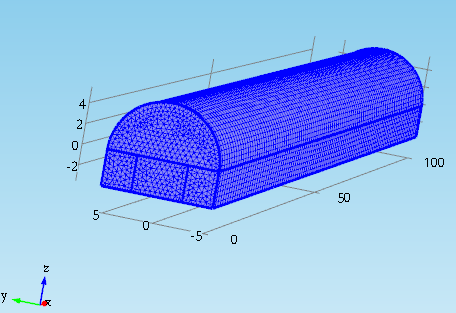Figure1. Computational model and mesh (m)

Assume the coal is uniform isotropy porous media and neglect the influence of gas and water. Assume that oxygen transports mainly through diffusion and convection, heat transports mainly through conduction and convection. Based on the heat and mass transport processes, principle of conservation of energy and principle of mass conservation, the mathematical model of coal spontaneous combustion is built.

$\rho \frac{\partial u}{\partial t}=\nabla \cdot\left[-p+\mu\left(\nabla u+(\nabla u)^{T}\right)\right]+F$       (1)

$\frac{\partial \rho}{\partial t}+\rho \nabla \cdot(u)=0$    (2)

$\frac{\partial c}{\partial t}+\nabla \cdot(-D \nabla c)+u \cdot \nabla c=R$    (3)

$\left(\rho C_{p}\right)_{\text {whole}} \frac{\partial T}{\partial t}+\rho C_{p} u \cdot \nabla T=\nabla \cdot\left(k_{\text {whole}} \nabla T\right)+Q$  (4)

Where $\left(\rho C_{p}\right)_{\text {whole}}$ is the equivalent heat capacity,

$\left(\rho C_{p}\right)_{\text {whole }}=(1-n) \rho_{c} c_{c}+n \rho C_{p}$ ,$k_{\text {whole}}$  is the equivalent heat conduction coefficient, $k_{\text {whole}}=(1-n) k_{c}+n k_{g}$

According to Equation (1)- Equation (4), the air flow of the coal is determined by the coupling of the velocity field and the temperature field. The oxygen consumption rate in the oxygen concentration field is controlled by temperature. The heat source of the temperature field is a function of oxygen concentration. Thus, coal spontaneous combustion is constantly pushed by the coupling of flow field, oxygen concentration field and the temperature field.

The characteristic parameters in the model such as oxygen consumption rate, heat release intensity and particle size influence function are obtained from the spontaneous combustion experiment . The permeability in the porous media depends on porosity. It is a function of porosity. The porosity of the loose top coal on site is 0.4. The porosity of the coal in the two sides is 0.15. Both are within the limit of 0-0.45. So the permeability expression can be obtained from reference . The values of main parameters in the model are shown in Table.1.

Table 1. Values of main parameters in the numerical simulation

 $\rho$ [kg/m3] 1.05 $\rho_{c}$ [kg/m3] 1350 $\mu$ [Pa s] 1.8e-05 $C_{\mathrm{p}}$ [J/(kg K)] 1005 $T_{0}$ [K] 298 $c_{c}$ [J/(kg K)] 1550 $n$ 0.4 $k_{c}$ [W/(m K)] 1.2 $C_{\varepsilon 1}$ 1.44 $k_{g}$ [W/(m K)] 0.029 $C_{\varepsilon 2}$ 1.92 $P_{0}$ [Pa] 1.2×105 $C_{\mu}$ 0.09 $D_{0}$ [m2/s] 10-5 $\sigma_{k}$ 1 $d v$ [mm] 5 $\sigma_{\varepsilon}$ 1.3 $d_{50}$ [mm] 7

The flow field of the coal depends on the airflow in the roadway. The flow velocity in the roadway is much higher than that in the coal. The flow in the roadway can be seen as fully-developed turbulent flow. Using Eq. (1) to describe the flow field in roadway is obviously inappropriate. In turbulent flow, the velocity of each particle generates pulsate randomly around the steady speed. Many researchers have studied  the  simulation of turbulent flow [12-13]. It’d be very hard to describe it by measurement or direct numerical simulation.  At present, most studies on turbulent flow velocity choose indirect method, for example experienced statistic based on statistical method. In terms of the computation of the roadway airflow field, the$k$ -$\mathcal{E}$turbulent model is chosen. The model is expressed in Eq. (5) to Eq. (8). $C_{\mu}$ ,$C_{\varepsilon 1}$ ,$C_{\varepsilon 2}$ ,$\sigma_{k}$ and$\sigma_{\varepsilon}$ are set according to reference , their values are listed in Tab. 1.

$\rho(u 2 \cdot \nabla) k 2=\nabla \cdot\left[\left(\mu+\frac{\mu_{T}}{\sigma_{k}}\right) \nabla k 2\right]+p_{k}-\rho \varepsilon$     (5)

$\rho(u 2 \cdot \nabla) \varepsilon=\nabla \cdot\left[\left(\mu+\frac{\mu_{T}}{\sigma_{\varepsilon}}\right) \nabla \varepsilon\right]+C_{\varepsilon 1} \frac{\varepsilon}{k 2}-C_{\varepsilon 2} \rho \frac{\varepsilon^{2}}{k 2}$    (6)

$\mu_{T}=\rho C_{\mu} \frac{k 2^{2}}{\varepsilon}$   (7)

$p_{k}=\mu_{T}\left[\nabla u 2:\left(\nabla u 2+(\nabla u 2)^{T}\right)-\frac{2}{3}(\nabla \cdot u 2)^{2}\right]-\frac{2}{3} \rho k 2 \nabla \cdot u 2$    (8)

The boundary conditions of the numerical simulation are as follows: The initial temperature of the loose top coal in the roadway is the primitive temperature of the surrounding rock 293K. The initial temperature of the airflow in the roadway is the average temperature of the roadway at normal work 300K. The volume concentration of the oxygen at the wind inlet of the roadway is 21%. The flow field of the roadway flows from the origin along the positive direction of X–axis. The ventilation of the roadway is 1500 m3/min.  So the wind velocity of the wind inlet is 1.4 m/s and the flow of the outlet is set as free flow. The top of the loose coal is adiabatic boundary. The walls of roadway are convection boundaries.

3. Results and Analyses of the Multi Field Coupling Numerical Simulation of Coal Spontanesous Combustion

## 2.png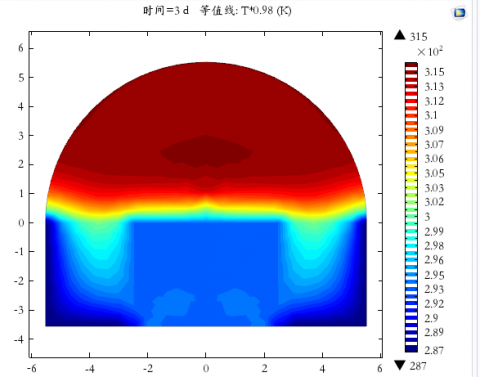Figure 2. Temperature distribution of the cross section at 15 meters away from the wind inlet of the roadway on the10th day (K)

The transient law of the flow field, the temperature field and oxygen concentration field at any moment from the top coal is broken massively to spontaneous combustion is acquired by COMSOL numerical simulation. According to the field observation data in reference, 10 days after the top coal  is broken massively, the temperature of the roadway surface at 15 m away from the wind inlet is about 30°C. At the same time, according to the results measured in bores drilling from the top of the roadway to the top coal, when the drilling depth is about 2 m, the temperature is 40 °C. Fig.2 shows the temperature distributions obtained by numerical simulation. The numerical simulation result is consistent with the field observation data.

Fig. 3 and Fig. 4 show the flow field distribution of the roadway and the top coal on the  10th day. The velocities  of the two flow fields are  basically the same as the site conditions. So the numerical simulation method used is feasible. In the simulation$k$ -$\mathcal{E}$ turbulent model is chosen to simulate the flow field of the roadway. The velocity of the roadway flow field is taken as the inflow boundary condition of the laminar flow in coal. As shown in Fig.4, along the length of the roadway, the velocities of the top coal near the entrance and the exit are relatively big. In terms of the cross section, the flow velocity of the loose coal near the top of the roadway is the largest, top area second, middle area third. Apart from the area near the roadway, the flow velocity of most areas of the top coal is within 0.0016 m/s -0.004 m/s, it is a spontaneous combustion zone.

## 3.pngFigure 3. Flow field distribution of the roadway on the 10th day (m/s)

## 4.png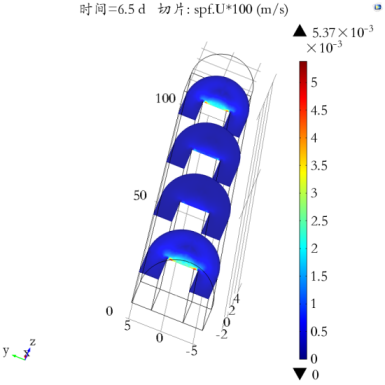Figure 4. Flow field distribution of coal on the 10th day (m/s)

3.1 Change of temperature field during spontaneous combustion of loose top coal in the roadway

After the top coal is broken massively, air in the roadway continuously percolates into loose coal at the top of the roadway and the relatively complete coal at  the two sides. According to Fig. 5-Fig. 7, as time goes by, the temperature of the loose top coal increases more and more quickly, while the temperatures of the two sides only increase a little. Affected by self-heating and heat convection, the temperature of the loose top coal rises slowly at the beginning, and then rises rapidly. After oxidation for 12 days, the highest temperature reaches 341K. After oxidation for 14 days, the highest temperature reaches 391K, higher than its threshold temperature 353K. Only three days later, the highest temperature reaches  its ignition point 650K. The threshold temperature and the ignition  point were obtained from the spontaneous combustion experiment.  It can be concluded that when temperature is higher than the threshold temperature, the temperature of the coal rises rapidly. It will be very hard to control. Thus, effective fire prevention measures should be taken before temperature reaches threshold temperature.

## 5.png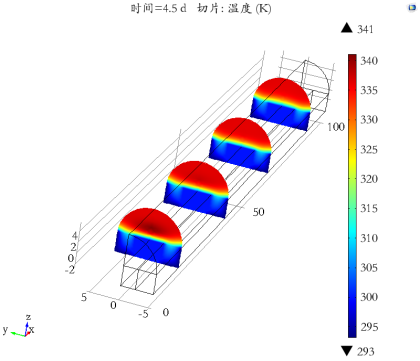Figure 5. Temperature distribution of cross sections on the 12th day (K)

## 6.png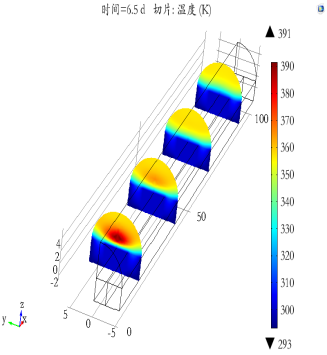Figure 6. Temperature distribution of cross sections on the 14th day (K)

## 7.png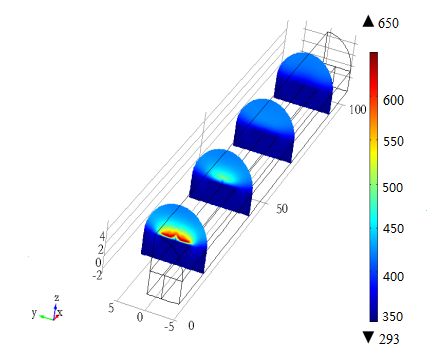Figure 7. Temperature distribution of cross sections on the 17th day (K)

According to Fig. 5-Fig. 7, the loose top coal near the roadway has the lowest temperature, top area second. High temperature zone is in the middle and low part of the top coal, very similar to the three zones of the goaf. The reason is that heat is hard to accumulate in the area near roadway because of heat convection. The oxygen concentration of the top area is low, so coal oxidation of this part is slow. While the air leakage intensity and oxygen concentration are moderate in the middle and low part, so this area is the high temperature zone. Meanwhile, after comparing Fig. 5-Fig. 7, we can see that the three temperature zones of the loose top coal are continuously changing over time. On the 12th day, 2 m-4 m above the top of the  roadway has the highest temperature. On the 14th day, 1.5 m-3.5 m above the top of the roadway has the highest temperature. On the 17th day, 0.5 m-1.8 m above the top of the  roadway has the highest temperature. It’s obvious that the high temperature zone is moving from the middle and low part to the low part of the loose coal, namely the wind inlet side. This change is also shown on the temperature distribution figures of y=0 section (Fig.8-Fig.10). That’s because the supplementary oxygen comes from air leakage. The oxygen concentration of the loose coal is decreasing with oxidation. As the oxygen supplementary of the top area is the hardest, it becomes the lowest oxygen concentration zone, and the zone grows larger and larger over time. Thus, the high temperature zone is continuously moving downwards.

## 8.pngFigure 8. Temperature distribution of y=0 section on the 12th day (K)

## 9.png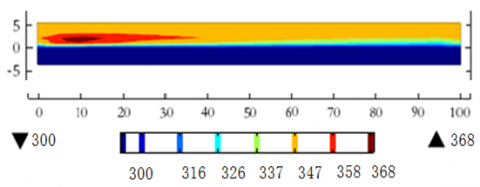Figure 9. Temperature distribution of y=0 section on the 14th day (K)

## 10.pngFigure 10. Temperature distribution of y=0 section on the 17th day (K)

Fig. 8-Fig. 10 show the temperature distribution on y=0 section. On the 12th day, the high temperature zone is from 2 m to 28 m on the  X-axis  and the core of it is at 11m. On the 14th day, the high temperature zone is from 2 m to 40 m on the X-axis and the core of it is at about 9 m. On the 17th day, high temperature zone is from 0 m to 50 m on the X-axis and the core of it is at about 5m. We can see that with the increase of temperature, the high temperature zone extends inward from the wind inlet along the  roadway continuously and the highest temperature point moves towards the wind inlet constantly.

3.2 Influencing factors of the spontaneous combustion of loose top coal in the roadway

It can be seen from 3.1 that the cause of the spontaneous combustion of the loose coal in the roadway is the oxygen percolated into the coal has exothermic reaction with the coal. Thus, the ventilation of the roadway and the crushing degree of the coal are the key factors of spontaneous combustion. The crushing degree of the coal depends on porosity. Based on the numerical simulation above, set the porosity of the loose coal as 0.2, 0.3 and 0.5 in sequence to consider the crushing degree’s influence on spontaneous combustion. Set the roadway ventilation as 1100 m3/min, 1700 m3/min and 1900 m3/min to explore its influence on spontaneous combustion.

Fig. 11 shows the change curves and fitting curves of time-highest temperature relations of the cross section during coal self-heating process. The cross section is 5 m away from the wind inlet. The ventilation is fixed and the porosity of coal is changed. It can be seen that the larger the porosity is, the quicker the temperature rises. That is to say, affected by the mine pressure, more broken the top coal is, the shorter the spontaneous combustion period is. That’s mainly because porosity influences the resistance of the airflow. The lager the porosity is, the smaller the resistance is. Larger porosity can accelerate heat convection.  But the heat capacity of air is small, huge air quantity can only bring down limited temperatures. So big porosity contributes to the self-heating of coal . In the model built in this paper, the time-highest temperature curves under different porosities can be expressed with  polynomials. R2 are larger than 0.99. When the porosity is 0.5, 0.4 and 0.3 respectively, the spontaneous combustion period is 9 days, 17 days and over 30 days. Spontaneous combustion won’t happen when porosity is less than 0.2.

## 11.png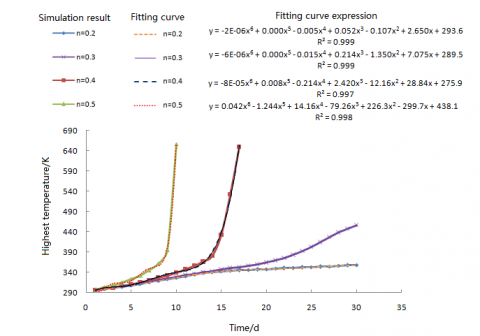Figure 11. Change curves and fitting curves of time-highest temperature relations under different porosities

## 12.png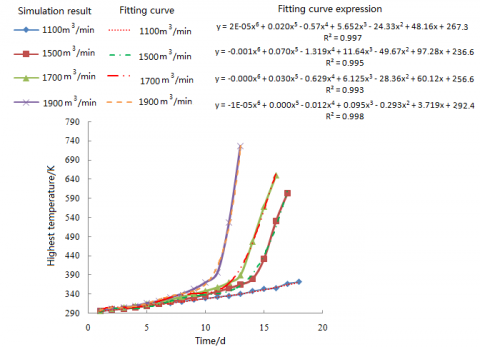Figure 12. Change curves and fitting curves of time-highest temperature relations under different ventilations

Fig. 12 shows  the change curves and fitting curves of time-highest temperature relations of the cross section during coal self-heating process. The cross section is 5 m away from the wind inlet. The porosity is fixed and roadway ventilation is changed. As the ventilation increases, the temperature rises quicker and spontaneous combustion period gets shorter. That’s because as the ventilaion increases, more oxygen percolates into coal, accelerates the oxidation. When ventilation is lower than a certain point, because of the convection of the roadway boundary and the thermal conductivity to the surrounding coal, low intense air leakage can’t give off enough heat to support temperature rising in the high temperature zone. In the model built in this paper, the time-highest temperature curves under different ventilations can be expressed with polynomials. R2 are  larger than 0.99. When the ventilation is 1900 m3/min, 1700 m3/min and 1500 m3/min respectively, the spontaneous combustion period is 12 days, 15 days and 17 days. Spontaneous combustion won’t happen when ventilation is less than 1100 m3/min.

4. Conclusions

(1)  Use $k$ -$\mathcal{E}$ turbulent model to describe the flow field of the roadway. Use laminar flow equation to describe the flow field of the loose top coal in the roadway. The calculated velocity of the roadway flow field is taken as the boundary condition of the flow field of the coal. This simulation method directly links the flow field of roadway and the spontaneous combustion of coal. The results of the numerical simulation can reflect the actual conditions of the two flow fields.

(2) In the process of coal self-heating, on cross sections, the loose top coal near the roadway has the lowest temperature, top area second. High temperature zone is in the middle and low part of the top coal. The high temperature zone gradually moves from the middle and low part  to the low part of the coal, namely the wind inlet side.

(3) In the process of coal self-heating, the high temperature zone on y=o section extends inward from the wind inlet along the roadway continuously and the highest temperature point moves towards the wind inlet constantly.

(4) Bigger ventilation and porosity can promote temperature rising and decrease the spontaneous combustion period. For the model built in this paper, the curves of time-highest temperature under different porosities and ventilations can be expressed with polynomials. Spontaneous combustion won’t happen when porosity is less than 0.2 or when ventilation is less than 1100 m3/min.

References

1. M. Yu, X. Chang, H. Jia and L. Lu, Analysis of Spontaneous Combustion “Three-zone” in Goaf based on Matlab, Journal of China Coal Society, vol.35, pp. 600-604, 2010. DOI: 10.13225/j.cnki.jccs.2010.04.024.

2. T. Chu, M. Yu, S. Yang and R. Pan, Numerical Simulation Analysis and Practice of Air Flow Field in Goaf based of  FLUENT, Journal of  Henan  Polytechnic University (Natural Science), vol.29, pp. 298-305, 2010. DOI: 10.16186 / j.cnki. 1673-9787. 2010.03.017.

3. Y. Zhou, Q. Meng, J. Li, B. Lin  and C. Xu, Research  on Numerical Simulation for Spontaneous Combustion Zone in Gob Area, Journal of China University of Mining &Technology, vol.43, pp.963-968, 2014. DOI: 10.13247/j.cnki.jcumt.000233.

4. K. Hooman and U. Maas, Theoretical Analysis of Coal Stockpile Self-heating, Fire Safety Journal, vol.67, pp.

107-112, 2014. DOI: 10.1016/j.firesaf.2014.05.011.

5. U. Green, Z. Aizenshtat, L. Metzger and H.  Cohen, Field and Laboratory Simulation Study of Hot Spots in Stockpiled Bituminous Coal, Energy and Fuels, vol.26, pp. 7230-7235, 2012. DOI: 10.1021/ef301474t.

6. M. Yu, L. Lu, X. Chang, Q. Deng and H. Li, Numerical Simulation Analysis on Spontaneous Combustion of Residual Coal in Top-coal Falling Region of Coal Drift, Journal of Disaster Prevention and Mitigation Engineering, vol.29, pp. 658-662, 2009. DOI: 10.3969/j.issn.1672-2132.2009.06.010.

7. D. Zhang, S. Yang, Q. Wang, L. Wang, H. Luo and G. liu, Numerical Simulation on the Spontaneous Combustion of Loose Coal in the Top-Coal Caving Region of Coal Drift, Journal of China University of Mining & Technology, vol.35, pp. 757-761, 2006. DOI: 10.3321/j.issn:1000-1964.2006.06.012.

8. H. Wen, J. Shi, X. Zhai, W. Sun, Y. Xu and L. Li, 3D Numerical Simulation on the Spontaneous Combustion of Loose coal in the Top-coal Caving Region of Full Seam  Roadway  with  Large  Section,  Journal  of Xi’an University of Science and  Technology,  vol.32,  pp. 537-542, 2012. DOI: 10.3969/j.issn.1672-9315.2012.05.001.

9. X. Liu and H. Han, Numerical Analysis of Dangerous Spontaneous Combustion Region Distribution  Feature of Coal Roadway Caving Zoo, Coal Technology, vol.33, pp.8-11, 2014. DOI: 10.1330/j.cnki.ct.2014.04.004.

10. H. Zhu and X. Liu, Investigation into Spontaneous Combustion Hazard and Numerical Simulation of Nitrogen Injection Fire Prevention Effects in the Roof Coal, Journal of China Coal Society, vol.37, pp. 1015- 1020, 2012. DOI: 10.13225 /j, cnki. jccs. 2012. 06.028.

11. J. Shi, Study on the Numerical Simulation  of  Loose Coal Spontaneous Combustion in Roadway, Ms. D. thesis, Xi’an University of Science and Technology, Shaanxi, Prov., 2012. DOI: 10.7666/d.d224274.

12. W. Chow and Y. Cao, Possible Application of Renormalization Group for Studying Turbulence in Engineering, International Journal of Heat and Technology, vol. 24, pp.79-87, 2006.

13. S. Li, W. Chow and S. Liu, Possibility of Simulating Turbulent Flow by the Lattice Boltzmann Method, International Journal of Heat and Technology, vol. 23, pp. 89-94, 2005.

14. D. C. Wilcox, Turbulence Modeling for CFD, 2nd ed., DCW Industries, 1998.

15. A. Ejlali, K. Hooman and B. Bearish, Effects  of  Porosity Changes on the Self-heating Characteristics of Coal Stockpiles, Journal of Porous Media, vol.15, pp. 869-876, 2012. DOI: 10.1615/JPorMedia.v15.i9.50.

16. B. Taraba, Z. Michalec, V. Michalcová, T. Blejchar, M. Bojko and M. Kozubková, CFD Simulations  of  the Effect of Wind on the Spontaneous Heating of Coal Stockpiles, Fuel, vol.118, pp. 107-112, 2014. DOI: 10.1016/j.fuel.2013.10.064.